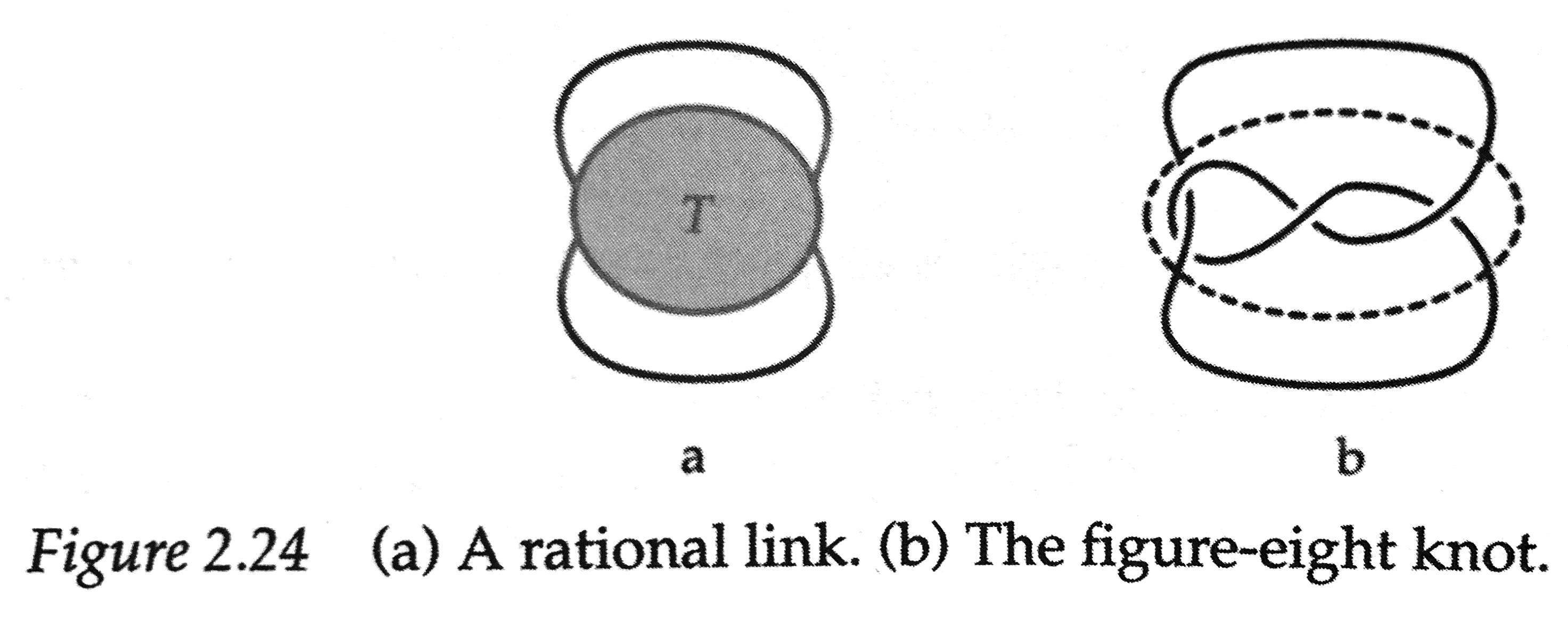$\newcommand{\identity}{\mathrm{id}} \newcommand{\notdivide}{{\not{\mid}}} \newcommand{\notsubset}{\not\subset} \newcommand{\lcm}{\operatorname{lcm}} \newcommand{\gf}{\operatorname{GF}} \newcommand{\inn}{\operatorname{Inn}} \newcommand{\aut}{\operatorname{Aut}} \newcommand{\Hom}{\operatorname{Hom}} \newcommand{\cis}{\operatorname{cis}} \newcommand{\chr}{\operatorname{char}} \newcommand{\Null}{\operatorname{Null}} \newcommand{\lt}{<} \newcommand{\gt}{>} \newcommand{\amp}{&}$

## Section1.3Tangle Arithmetic

So far, our strategy for understanding the structure of rational tangles has been to see them as constructed through a series of geometric operations, twists and rotations, applied to an empty tangle. But that doesn't give us a very friendly way of determining the tangle number for a rational tangle, nor (especially!) for going the opposite direction. Our next goal is to find a more systematic way of determining the tangle number of a rational tangle, which is also capable of -- just as systematically, we hope -- determining the rational tangle associated with a given rational number. This system is built on a new, less geometric, more arithmetic vision of how rational tangles may be defined.

### Subsection1.3.1Arithmetic, not Geometry

Rather than thinking about rational tangles as the result of applying the geometric operations of twisting and rotating ($T$ and $R$) to an empty tangle, what we want instead is a way of thinking about these as operations of arithmetic. Doing that will require us to define what it means to:

1. Add two tangles, $G + H\text{,}$ and
2. Multiply two tangles, $G*H\text{,}$

both in a way that aligns with our original geometric notions and which have the effects that we expect on tangle number.

### Subsection1.3.2Flips and Flypes: The Invisible Arithmetic

Addition and multiplication are the tangle operations that "matter" in arithmetic. Indeed, we now define rational tangles as those tangles which can be built through addition and multiplication of the simple twists $$ and $[-1]$ beginning from an empty tangle.

But since the addition and multiplication are not in general commutative on tangles (that is, it's not always true that $G + H \simeq H + G$ or that $G*H \simeq H*G$), we now introduce a new set of tangle operations that don't make a difference to arithmetic, but they do help us get around the lack of commutativity by permitting us to flype left- and top-twists to turn them all into right- and bottom-twists only.

### Subsection1.3.3Continued Fractions: The Bridge Between Tangle and Number

Since we now know that all rational tangles can be built solely using right- and bottom-twists, we can determine the arithmetic effects of each of those basic operations. If the tangle number of $G$ is $x \in\mathbb{Q}\text{,}$ then

1. Making a twist on the right adds to the tangle number: \begin{equation*} G + [n] \rightsquigarrow x + n \end{equation*} and
2. Making a twist on the bottom conjugates that addition with rotation, i.e. the reciprocal. In other words, making a bottom twist is the same as taking a reciprocal, then making a right twist (adding), then taking the reciprocal again: \begin{equation*} G* \frac{1}{[n]} \simeq \frac{1}{[n]+\frac{1}{G}} \rightsquigarrow \frac{1}{n+\frac{1}{x}}. \end{equation*}

This gives us the key result of this section: that rational tangles, as well as their tangle numbers, each have (identical) representations as continued fractions.

### Subsection1.3.4Using Continued Fractions to Convert Tangles and Numbers

Theorem 1.3.1 shows that the (canonical) continued fraction representation is our bridge between rational tangles and rational numbers. Combined with an algorithm that we can use to compute the canonical continued fraction representation for any $x\in\mathbb{Q}\text{,}$ this gives us all we need to travel between worlds.

### Subsection1.3.5Exercises

###### 1

Sketch the rational tangles $T_1,T_2,T_3$ whose tangle numbers (fractions) are $F(T_1)=4/5\text{,}$ $F(T_2)=-5/8\text{,}$ and $F(T_3)=9/2\text{.}$

###### 2

For each of the following knots $K\text{,}$ find and sketch a rational tangle $T$ for which $K = N(T)$ is the numerator closure of $T\text{.}$ Adams' sketch in the diagram below can be an inspiration:(a) $K = 3_1\text{,}$ the trefoil. (b) $K = 4_1\text{,}$ the figure-eight. (c) $K = 6_3\text{.}$

###### 3

Determine the tangle number for each of the tangles you found in Exercise 1.3.5.2.

###### 4

Refer to the two equivalent tangles from Exercise 1.2.4.2, which Adams notates as

\begin{equation*} T_1 = -2\; 3\; 2 = \bigl[ , , [-2] \bigr] \qquad {\rm and} \qquad T_2 = 3\; -2 \; 3 = \bigl[ , [-2],  \bigr]. \end{equation*}

Verify that the tangle numbers (fractions) agree, i.e., that $F(T_1) = F(T_2).$

###### 5

([KL] Lem. 5.3 and Lem. 6.3) Let $\frac{p}{q} = [a_1,a_2,a_3,\ldots,a_n]$ be a rational number written as a continued fraction.

1. Prove that $[0,a_1,a_2,a_3,\ldots,a_n] = \frac{q}{p}$ represents its reciprocal.
2. What relationship exists between the rational tangles \begin{equation*} T_1 = \bigl[ [a_1],[a_2],[a_3],\ldots,[a_n]\bigr] \qquad {\rm and} \qquad T_2 = \bigl[ , [a_1],[a_2],[a_3],\ldots,[a_n]\bigr]? \end{equation*} Explain the relationship using pictures, and in words explain why this relationship is not surprising.